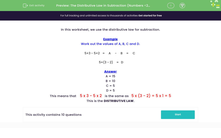# The Distributive Law in Subtraction (Numbers <20)

In this worksheet, students use the distributive law in subtraction.Key stage:  KS 3

Curriculum topic:   Number

Curriculum subtopic:   Use Relationships Between Operations

Difficulty level:#### Worksheet Overview

In this worksheet, we use the distributive law for subtraction.

Example

Work out the values of A, B, C and D.

5×3 - 5×2 =
 A
-
 B
=
 C

5×(3 - 2) =
 D

A = 15
B = 10
C = 5
D = 5

This means that    5 x 3 - 5 x 2   is the same as   5 x (3 - 2) = 5 x 1 = 5

This is the DISTRIBUTIVE LAW.

### What is EdPlace?

We're your National Curriculum aligned online education content provider helping each child succeed in English, maths and science from year 1 to GCSE. With an EdPlace account you’ll be able to track and measure progress, helping each child achieve their best. We build confidence and attainment by personalising each child’s learning at a level that suits them.

Get started